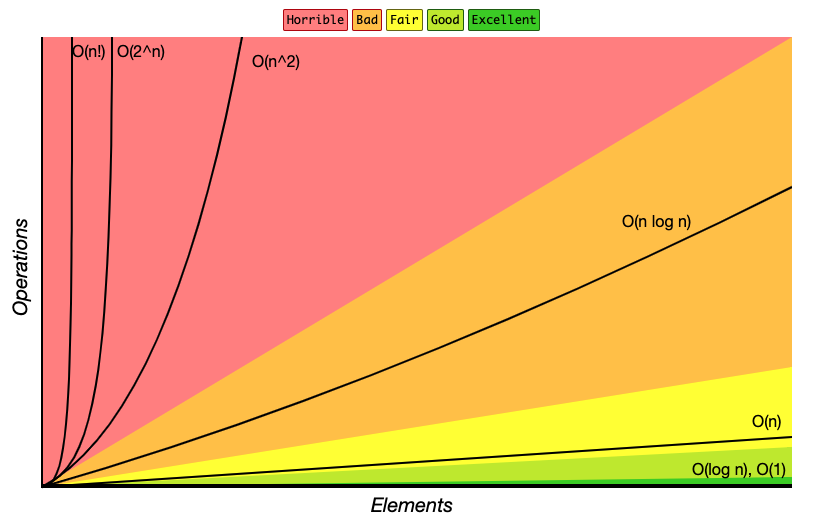Here is a handbook to help us better understanding algorithm.

Program = Data Structure (DS) + Algorithm

## Algorithm Analysis

It’s very common for programmers to compare their programs or algorithms with one another. When two programs solve the same problem but look different, is one program better than the other?

• Time
• Space

### Analysis Method

Notations:

• Big O (The worst)
• Big Omega (The best)
• Big Delta (The range between the worst and the best)

We use the “Big-O Notation” to expression complexity of a algorithm.

Here is some common algorithm complexities:

• O(1)
• O(log n)
• O(n)
• O(n log n)
• O(n^2)
• O(2^n)
• O(n!)

Big-O Complexity Chart## Data Structure

• Linear
• Array (List)
• Queue
• Simple Queue
• Circular Queue
• Priority Queue
• Double Ended Queue (Deque)
• Multi-level Feedback Queue，MLFQ
• Deque
• Stack
• Non-linear
• Heap
• Binary Heap
• Max Heap
• Min Heap
• Tree
• Binary Tree
• Full Binary Tree
• Perfect Binary Tree
• Complete Binary Tree
• Balanced Binary Tree
• Binary Search Tree
• AVL Tree
• Disjoint-set
• Graph
• Directed Graph
• Directed Acyclic Graph
• Indirected Graph

## Algorithm Design

### Algorithm Strategy

Algorithm strategy can also be called Algorithm Paradigm, it’s a general method or template for designing and solving a class of problems. Each algorithm strategy has its own design ideas and problem-solving steps.

• Brute Force Enumeration
• Classic Applications
• Selection Sort
• Bubble Sort
• Linear Search
• Greedy Algorithm
• Features
• It may not find the optimal solution
• Classic Applications
• Making change using the fewest coins
• Divide and Conquer
• Classic Problems
• Quick Sort
• Merge Sort
• Binary Search
• Backtracking
• Classic Problems
• Depth First Search (DFS)
• N Queens Problem
• Dynamic Programming (DP)
• Features
• Optimal Substructure
• Overlapping Sub-Problem
• Approaches
• Top-down Approach
• Down-Top Approach
• Classic Problems
• Fibonacci Sequence
• Making change using the fewest coins
• Longest Common Subsequence (LCS)
• Shortest Common Supersequence (SCS)
• Longest Increasing Subsequence (LIS)
• The Levenshtein distance (Edit Distance)
• Sliding Window
• Features
• Consecutive elements
• Calculate maximum value
• Classic Problems
• Max sum of any k consecutive elements in the array
• Longest substring without repeating characters
• Finite State Machine (FSM)
• Features
• The sub-problem can be divided to different states
• Helps to avoid so many nested if-else statements in your code and make the code much simpler
• Steps
• Draw the finite state machine diagram
• Identify all types of input data
• Identify all possible states for the FSM
• Identify the valid transitions between states for given inputs
• Follow the FSM diagram to implement the algorithm
• Classic Problems

### Algorithm Implementation

We should understand `Strategy != Algorithm`, algorithms are just means of implementing strategies. Recursion is an implementation of the algorithm strategies.